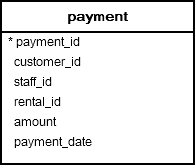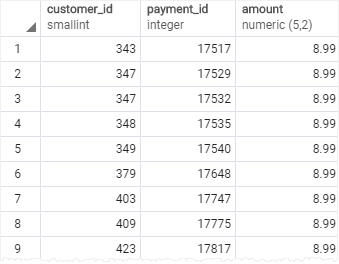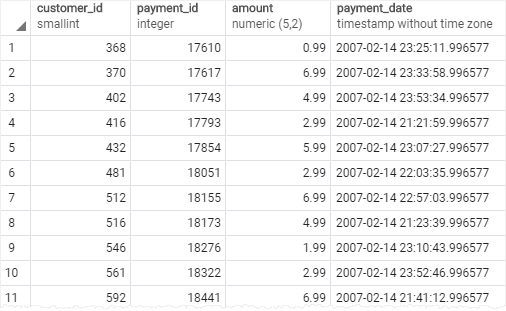# PostgreSQL Tutorial: BETWEEN

August 1, 2023

Summary: in this tutorial, you will learn how to use the PostgreSQL BETWEEN operator to match a value against a range of values.

## Introduction to the PostgreSQL BETWEEN operator

You use the `BETWEEN` operator to match a value against a range of values. The following illustrates the syntax of the `BETWEEN` operator:

``````value BETWEEN low AND high;
``````

If the `value` is greater than or equal to the `low` value and less than or equal to the `high` value, the expression returns true, otherwise, it returns false.

You can rewrite the `BETWEEN` operator by using the greater than or equal ( `>=`) or less than or equal ( `<=`) operators like this:

``````value >= low and value <= high
``````

If you want to check if a value is out of a range, you combine the `NOT` operator with the `BETWEEN` operator as follows:

``````value NOT BETWEEN low AND high;
``````

The following expression is equivalent to the expression that uses the `NOT` and `BETWEEN` operators:

``````value < low OR value > high
``````

You often use the `BETWEEN `operator in the WHERE clause of a SELECT, INSERT, UPDATE or DELETE statement.

## PostgreSQL BETWEEN operator examples

Let’s take a look at the `payment `table in the sample database.The following query use the BETWEEN operator to select payments whose amount is between 8 and 9 (USD):

``````SELECT
customer_id,
payment_id,
amount
FROM
payment
WHERE
amount BETWEEN 8 AND 9;
``````To get payments whose amount is not in the range of 8 and 9, you use the following query:

``````SELECT
customer_id,
payment_id,
amount
FROM
payment
WHERE
amount NOT BETWEEN 8 AND 9;
``````If you want to check a value against of date ranges, you should use the literal date in ISO 8601 format i.e., YYYY-MM-DD. For example, to get the payment whose payment date is between `2007-02-07` and `2007-02-15`, you use the following query:

``````SELECT
customer_id,
payment_id,
amount,
payment_date
FROM
payment
WHERE
payment_date BETWEEN '2007-02-07' AND '2007-02-15';
``````In this tutorial, you have learned how to use PostgreSQL `BETWEEN `operator to select a value that is in a range of values.